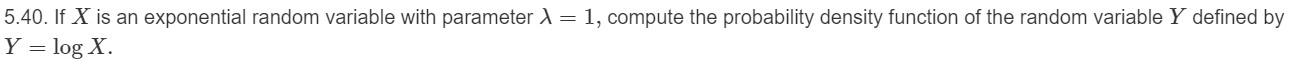# 5.40. If X is an exponential random variable with parameter Y log X = 1, compute the probability density function of the random variable Y defined by

Question

5.help_outlineImage Transcriptionclose5.40. If X is an exponential random variable with parameter Y log X = 1, compute the probability density function of the random variable Y defined by fullscreen

### Want to see this answer and more?

Experts are waiting 24/7 to provide step-by-step solutions in as fast as 30 minutes!*

*Response times vary by subject and question complexity. Median response time is 34 minutes and may be longer for new subjects.
Tagged in
MathStatistics

### Other